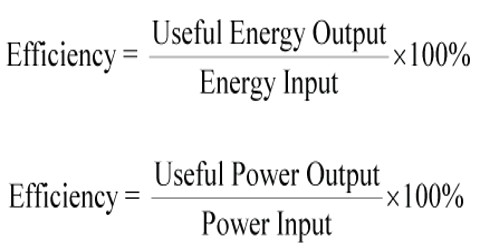# Efficiency in Energy

Efficiency Energy

When we get work from a machine or a body that work is smaller than the energy supplied to that machine or body to do work. It is not only true for machine, but also true in our practical life whereat a part of work is utilized than the supplied energy. Rest of energy is lost. In case of engine this dissipation of energy is used as friction, to warm up the engine etc. This dissipation cannot be stopped completely, but by applying different technologies this dissipation can be reduced.

In this case, equation of energy is equal to supplied energy = available effective energy + used energy by other means.

Definition: The efficiency of a machine is defined as the ratio of the output energy to input or supplied energy. It is denoted by η (eta)

Efficiency η = output energy / input energy

Efficiency can be expressed in percentage.

Let E1 be the energy supplied and E2 be the dissipation of energy, then

η = (E1 – E2)/E1 = (1 – E2/E1) x 100%

No machine has 100% efficiency. For example, efficiency of a machine is 80% means that by applying 100 units of energy we can get 80 units of useful energy and the test 20 units will be lost.